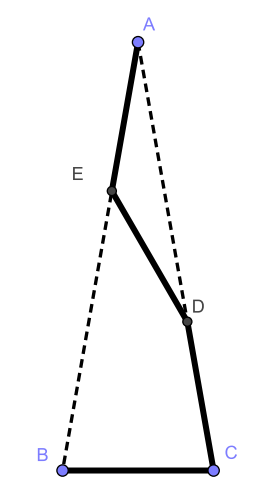# Geometry Questions

I’m studying for my Geometry class and need an explanation.

I will upload what I’ve worked.

1. A light beam is reflected (angle of incidence = angle of reflection) against three flat mirrors according to the figure. Determine the angle β expressed in the angle α.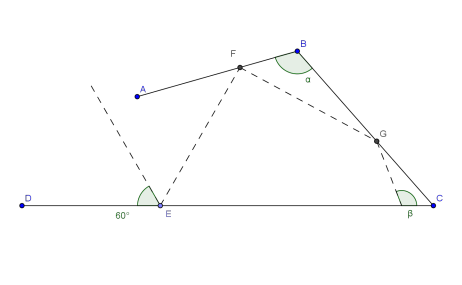2. ABCD is a convex quadrilateral. This means that the distance between two is arbitrarily chosen points in the quadrilateral are inside the ABCD. In a concave quadrilateral can a piece of the line lie outside the area. Each side of the quadrilateral is divided into three equal parts, which gives rise to to eight new points E, F, G, H, I, J, K, L. By connecting these points in pairs (see figure) four new lines are created that intersect at the points M, N, O, P. What can you say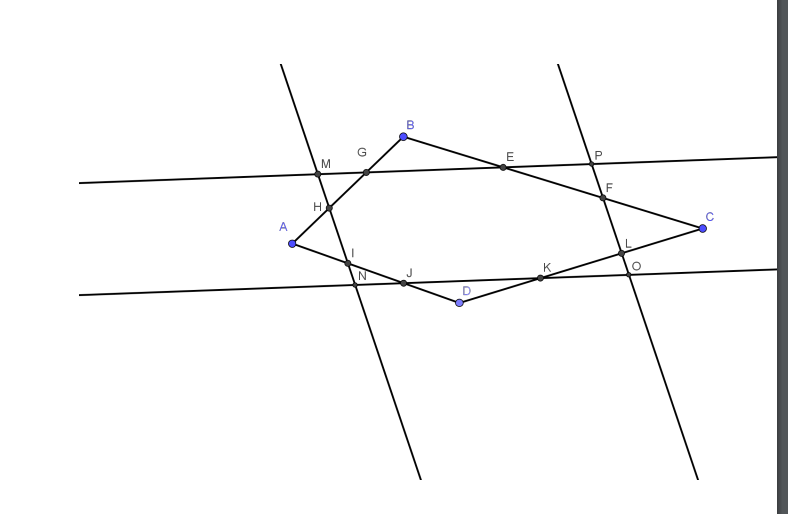3. Let the ABCD be a parallelogram. From corner A you draw the line AN perpendicular to

CD and the distance AM perpendicular to CB. Point N lies on the line CD and point M is on the line CB, see figure. Show that the triangles MAN and ABC are uniform. Management:

Use theorems: In a circle, peripheral angles that stand on the same arc are equal.

The peripheral angle of a semicircle is right.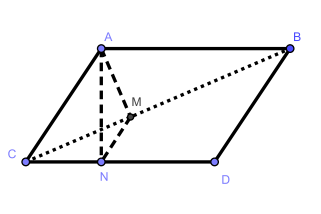4. A right-angled triangle, T has catheters a and b and hypotenuse c. Three new triangles, all

uniform with the original, is created as follows: the first new triangle, T1, is obtained by multiplying all the sides of T by the factor a. In the same way, T2 and T3 are obtained by to multiply the sides in T by b and c respectively. Now puzzle these three new triangles together

to a rectangle. Show with a figure how you did. Enter the sides of the rectangle. What are you drawing for a Conclusion?

5. Two well-known trigonometric formulas are

sin 2α = 2 sin α cos α,

cos 2α = cos2 α – sin2 α

Show these formulas when α is an acute angle.

Lead: Consider an isosceles triangle with apex angle 2α.

6. A circle touches three of the sides of a rectangle and cuts the fourth side into three equal parts. Determine the relationship between the sides of the rectangle.

7. In a circle with radius r, an arc of a circle with a center angle of 90 degrees is dashed in the figure below. It is folded over (downwards) and this arc of a circle is solid in the figure. Then one is entered smaller circle symmetrically in the figure. Two equal claw-like areas are then formed. Calculate area of these.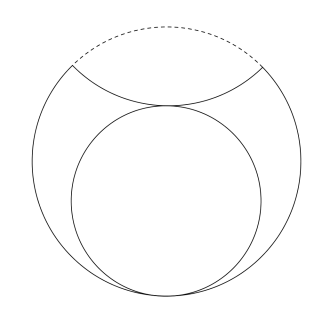8. Two radii in a circle form 45 degrees with each other. A chord is divided by these radii into three equal parts. Determine the relationship between the chord and the diameter of the circle.

9. An equilateral triangle ABC has side 1. A point D has the distance 2 to C and the distance

3/2 to A. Determine the maximum and minimum value of the section BD. Note that you must answer exactly. Utilize the trigonometric relationships such as cos (α + β) = cos α cos β –

sin α sin β.

10. The three bisectrices in a triangle always intersect at the midpoint, 0, of the inscribed

the circle. Let AA1 be the bisector of the angle A in ∆ABC where A1 lies on the side BC (see

figure). Show that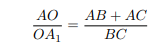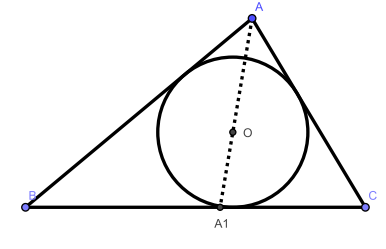11. In a right-angled triangle, the hypotenuse is divided into two parts A and B of the inscribed circles tangent point. Show that the area of the triangle is AB.

12. Two circles with radii of 13 cm and 1 cm touch each other on the inside. A chord in

the larger circle is tangent to the smaller circle and forms an angle of 30 degrees with the line

through the centers of the circles. Determine the length of the cord. See figure.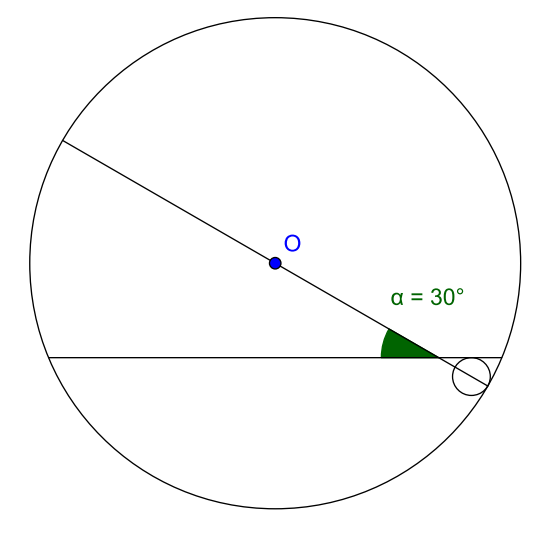13. In a square with side a, a portrait of a bee has been drawn (see figure).

What is the radius of the circle depicting the head? The wings are bounded by two semicircles

with diameter a and a quarter circle with radius a and center in the upper right corner. The

the three arcs of the circle intersect at three points and the small circle passes through these three points. The small circle depicts the hind body. The large circle (head) touches two

of the sides of the square and the two semicircles.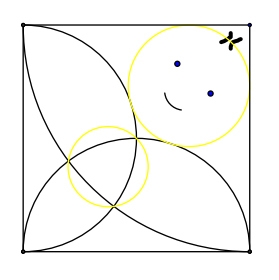14. A mirror has broken, see figure. The EF GH mirror is a square and the ABCD is also one

square. In all four triangles that make up the frame, one catheter is twice as long the other, AF = 2AE and so on. For the crack EIJKLG it applies that EI = 12, IJ = 6, JK = 8, KL = 3, LG = 7. The segments in the crack are perpendicular to each other so EI⊥IJ, IJ⊥JK and so on. Determine the side of the square frame AB.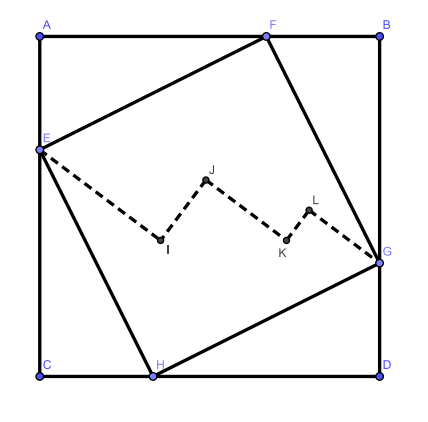15. Four equally long rods (solid lines in the figure) are laid out along an isosceles triangle (see figure). How large is the angle at A?